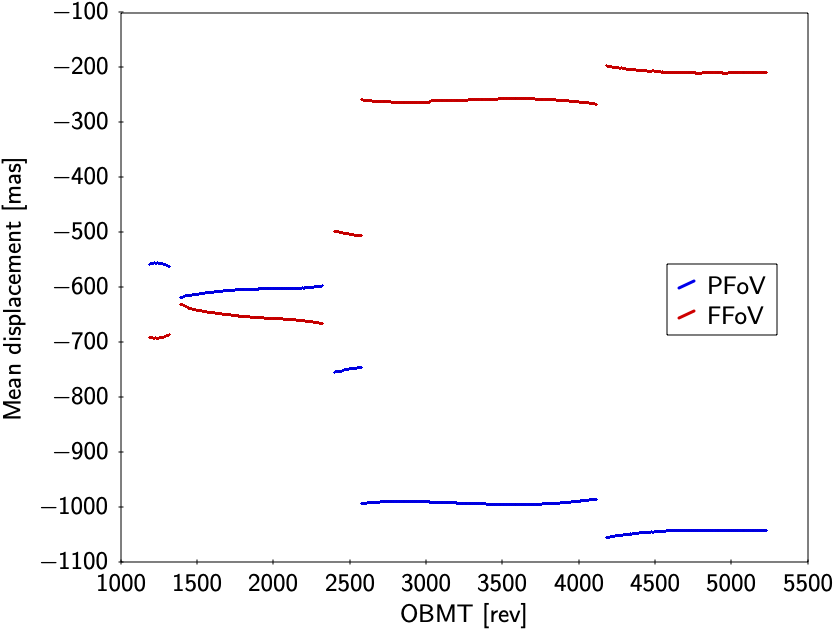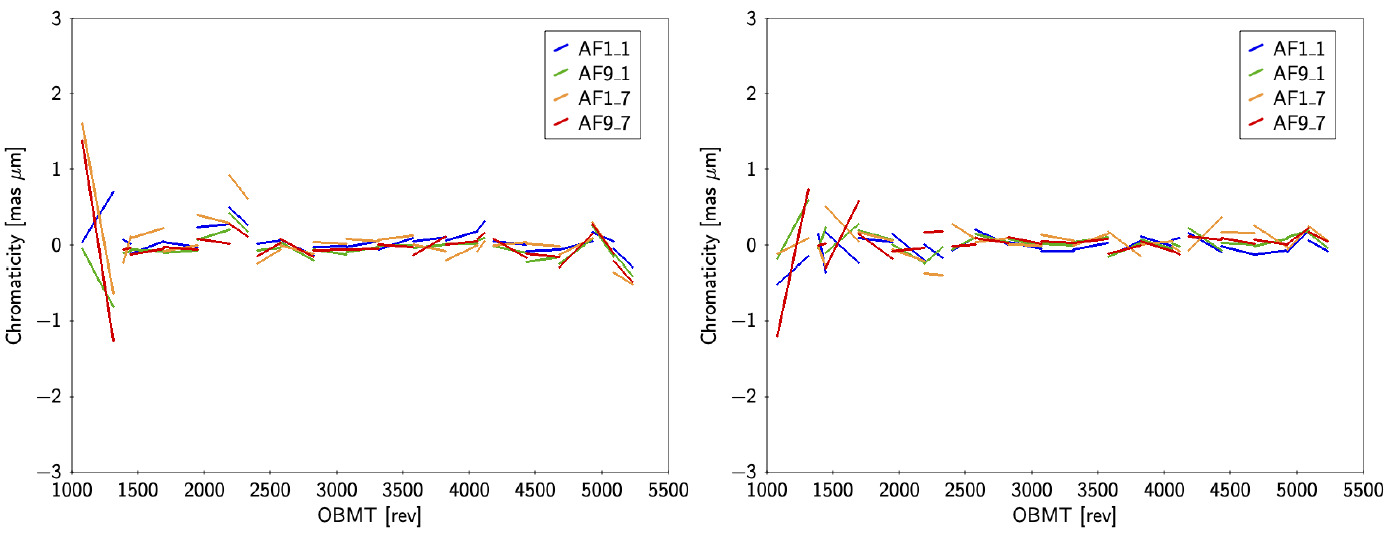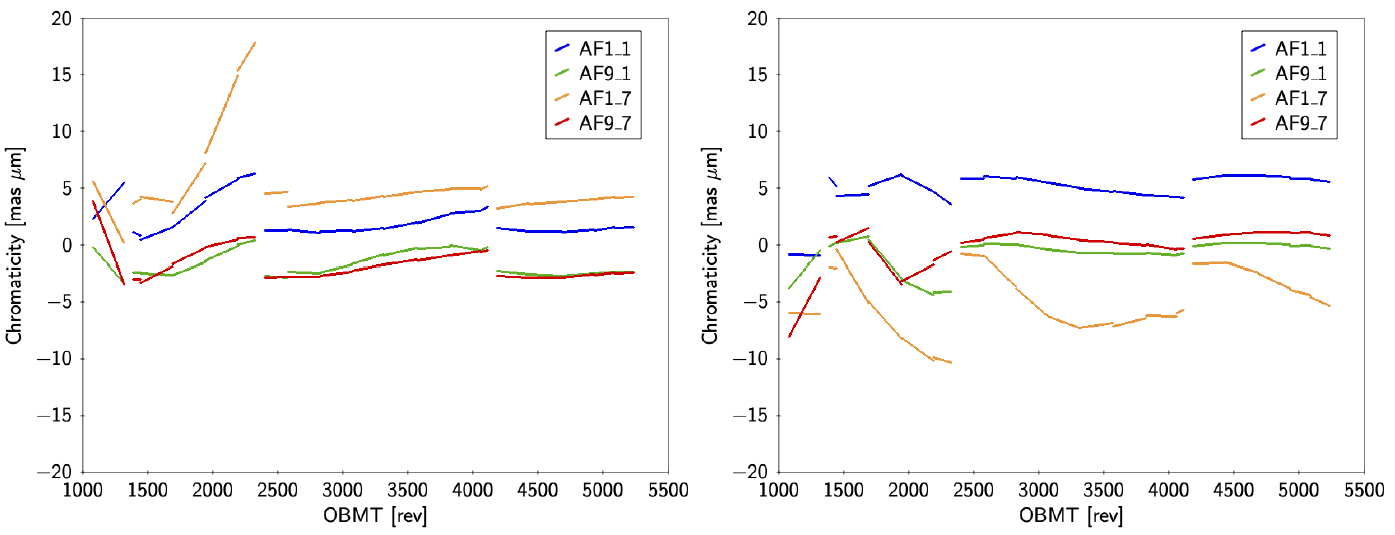# 4.5.5 Geometric calibration verification

Author(s): Alex Bombrun

The calibration model is described in Section 4.3.6. This model is more complex than the original proposal to take into account effects not corrected yet in the PSF/LSF calibration Section 3.3.5. The validation of the calibration model is strongly tied to the analysis of AGIS residuals, i.e. one looks for any dependencies in the residuals that can be compensated by some effect in the calibration model. Besides the analysis of the residuals one can also plot the calibration parameters and check that they are consistent with the instrument history. An example of this is given in Figure 4.24.Figure 4.24: Large-scale AL geometric calibration in the two fields of view interpreted in terms of (top) the mean displacement of the origin η=0; (middle) the mean rotation of the field around its optical axis; and (bottom) the mean effective focal length. Discontinuities are seen at the times of decontamination and refocusing. The differential displacement between the preceding and following field in the left diagram corresponds to a change in the basic angle; however, the jumps at OBMT 1330, 2340, and 4120 rev are partly not physical, caused by the difficulty to track the fringes of the basic-angle monitor (BAM) metrology system across large data gaps (see Table 4.2).

In particular the calibration model contains chromatic corrections (see Section 4.3.4) that were critical to get a good astrometric solution. In Figure 4.25 and Figure 4.26 one can see the time evolution of the chromatic corrections for selected CCDs and per field of view as computed in AGIS for window class 1 observations. The first figure is for the sources where the available colour information could be used to select the appropriate LSF or PSF to fit in the image parameter determination stage of the pre-processing; the second figure is for the sources without such colour information, where a default colour was assumed for the image parameter determination. Comparison of the two figures shows that the colour-dependent LSF/PSF calibration in IDU, based on an earlier AGIS solution, successfully eliminated most of the chromaticity, thus demonstrating the validity of the AGIS-PhotPipe-IDU loop processing described in Section 4.4.6.Figure 4.25: Chromaticity calibration in AGIS for window class WC1 in the preceding field of view (left) and following field of view (right). This is the calibration for sources with good colour information available at the IDU pre-processing stage, and for which image parameters could be determined by fitting the LSF or PSF appropriate to the colour of the source.Figure 4.26: Chromaticity calibration in AGIS for window class WC1 in the preceding field of view (left) and following field of view (right). This is the calibration for sources that did not have a reliable colour at the time of the IDU pre-processing: the image parameters were determined by fitting the LSF or PSF for the default colour, which resulting in a much larger residual chromaticity in AGIS. This chromaticity calibration was subsequently used to determine the pseudocolours of the sources.

Besides residual analysis and plotting of the fitted calibration values, one can also compare the geometric calibration model to the BAM metrology. In particular, as we have introduced break points in the calibration model corresponding to the times of some of the largest BAM jumps, one can compare the jumps derived from the calibration model with the ones introduced in the basic angle corrector. From the current state of this analysis, it seems that a relatively large fraction of the BAM jumps are not astrometric jumps as they are fully compensated by a jump of opposite amplitude in the calibration model.